Application of one-time Signatures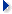One-time signature basics
Previous: Application of one-time Signatures Up: Application of one-time Signatures Next: The current implementation

One-time signature scheme was first introduced by Lamport [DH76, Lam79] and more efficient schemes have been proposed since then [Mer87, Mer89].

We will assume here that the hash function h produces l bits and the message digests to be signed are n-bit long. The first step involved is the creation of a key-pair which will be used to sign a file only once; for this purpose, two arraysandare generated. The first one containstruly random l-bit-numbers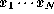, and the second contains the hash values of these numbers, that is,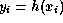. By definition the public key is:.

The second step is to compute the signature of a file f whose hash is noted. The signature of f is simply an array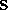whose N components are:where the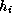's are the binary digits ofand the's the binary digits of a checksum. This checksum prevents attacks in which an opponent could produce a file f' such that all the `1' inare also inbut some `0' inhave been replaced by `1'. Once the signature is generated, the private keyshould be destroyed.

Given f,and K, verifying the signature implies: compute, c, construct an arraysuch that:and check that.

Fabien A.P. Petitcolas, Computer Laboratory, University of Cambridge